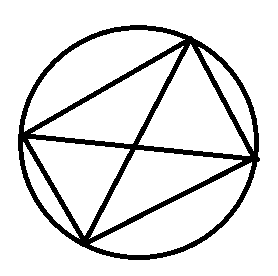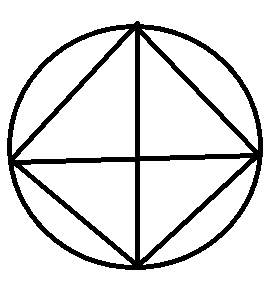QUESTION

# Draw a circle and any two of its diameters. If you join the ends of these diameters, what is the figure obtained? What figure is obtained if the diameters are perpendicular to each other? How do you check your answer?

Hint: A circle is a shape consisting of all points in a plane that are a given distance from a given point, the centre; equivalently it is the curve traced out by a point that moves in a plane so that its distance from a given point is constant. The distance between any point of the circle and the centre is called the radius.

Complete Step-by-Step solution:
Specifically, a circle is a simple closed curve that divides the plane into two regions: an interior and an exterior. In everyday use, the term "circle" may be used interchangeably to refer to either the boundary of the figure, or to the whole figure including its interior; in strict technical usage, the circle is only the boundary and the whole figure is called a disc.
As mentioned in the question, we have to find the figures that would be obtained on joining the ends of the diameters.
When the diameters are not perpendicular to each other, then the figure that we will get would be as followsNow, when the diameters are not perpendicular to each other, then the figure that we will get would be as followsOn observing the above figure, we can observe that all the angles of the figure are ${{90}^{\circ }}$ as the diameters subtend the right angle at the boundary of the circle.
Another thing that we observe is that both the diameters are perpendicular to each other as well as they bisect each other at right angles.
Hence, the figure here that we are getting is a square.
Note: The students can make an error if they don’t know about the property of diameters of a circle as without knowing them, one could not solve this question correctly.